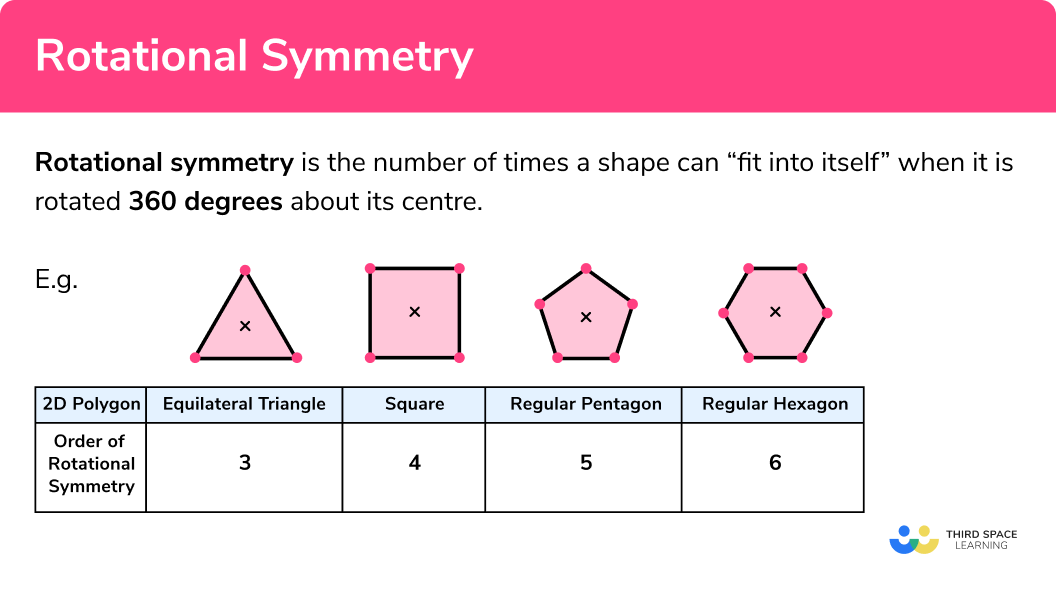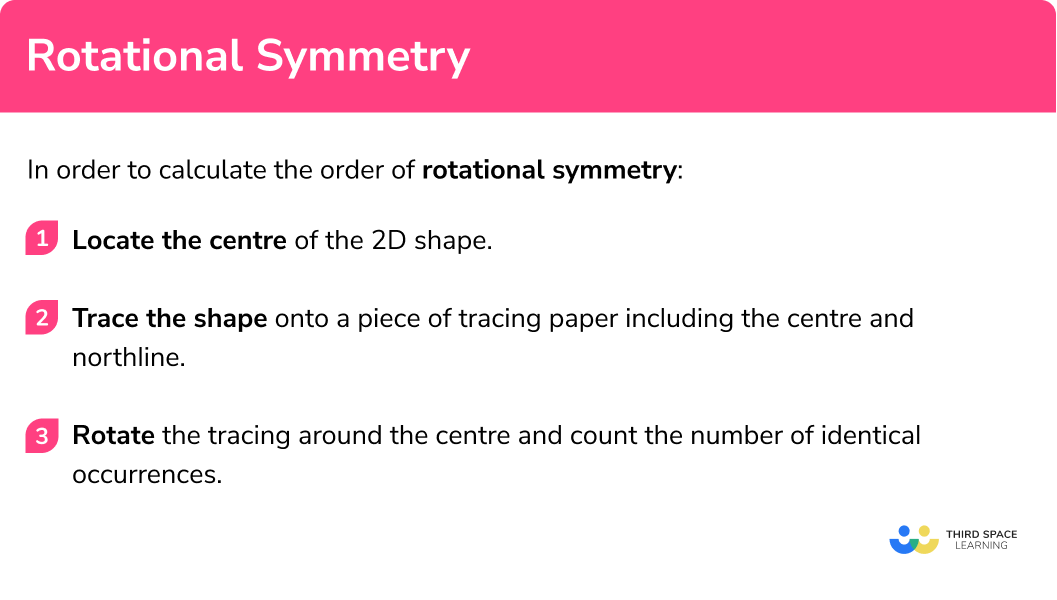# Rotational Symmetry

Here we will learn about rotational symmetry, including rotational symmetry within polygons, angle properties, and symmetry of different line graphs.

There are also rotational symmetry worksheets based on Edexcel, AQA and OCR exam questions, along with further guidance on where to go next if you’re still stuck.

## What is rotational symmetry?

Rotational symmetry is the number of times a shape can “fit into itself” when it is rotated 360 degrees about its centre.

E.g.

A rectangle has a rotational symmetry of order 2 shown below where one vertex is highlighted with a circle and the centre of the shape is indicated with an ‘x’. In the diagram, the shape looks identical in two orientations and so the rotational symmetry of the rectangle is 2.

To calculate the order of rotational symmetry of a shape, you need to locate the centre of the shape.

If the polygon has an even number of sides, this can be done by joining the diagonals. If the polygon has an odd number of sides, this can be done by joining each vertex to the midpoint of the opposing side.

You then rotate the shape 360 degrees around the centre and see how many times the shape looks exactly like the original.

### What is rotational symmetry?### Rotational symmetry in regular shapes

Many 2D shapes have a rotational symmetry. If a shape is rotated around its centre and the shape returns to the original position without it “fitting into itself”, then the shape is described to have no rotational symmetry. Irregular shapes tend to have no rotational symmetry. Regular polygons have the same number of sides as their rotational symmetry.

A reason why regular shapes have the same number of sides as their rotational symmetry is due to the angles and side lengths within the shape being the same.

### Rotational symmetry in irregular shapes

As soon as the angles in two-dimensional shapes change from their equal property, the order of rotational symmetry changes.

Let’s look at different shapes (specifically quadrilaterals) and their order of rotational symmetry.

The kite is interesting because it may appear to have rotational symmetry due to it having a line of symmetry. However if the shape is rotated around its centre, it returns back to the original orientation without it fitting into itself again so the order of rotational symmetry for a kite is 1 .  We can also state that any shape with rotational symmetry order 1 has “no rotational symmetry”.

This is also true for any other quadrilateral that is not a square, rectangle, parallelogram or rhombus.

### Rotational symmetry in graphs

We don’t stop at shapes when we look at rotational symmetry. We can also consider rotational symmetry with different types of graphs.

E.g.

Below is the graph of the equation y=x.

Given that the line extends in both directions beyond the axes drawn above, we can use the origin as a centre of rotation. If we rotate the line 180 degrees about the origin, we will get exactly the same line. Continuing this rotation all the way through 360^o we get back to the original.

So the line y=x has an order of rotation of 2 .

### Rotational symmetry in circles

A regular hexagon has an order of rotation of 6 , an octagon has an order of rotation of 8 , and a dodecagon has an order of rotation of 12 . But what about a circle?

A circle can be rotated around its centre and the shape will remain identical as the radius is the same for every point on the circumference of the circle.

This means that the order of rotational symmetry for a circle is infinite.

## How to calculate the order of rotational symmetry

In order to calculate the order of rotational symmetry:

1. Locate the centre of the 2D shape.
2. Trace the shape onto a piece of tracing paper including the centre and north line.
3. Rotate the tracing around the centre and count the number of identical occurrences.

### Explain how to calculate the order of rotational symmetry## Rotational symmetry examples

### Example 1: the isosceles triangle

Calculate the order of rotation for the isosceles triangle below:

1. Locate the centre of the 2D shape.

Draw a small x in the centre of the triangle (draw a line from each vertex to the midpoint of the line opposite).

2Trace the shape onto a piece of tracing paper including the centre and north line.

Here we use tracing paper to trace the shape including the centre of the shape and an upwards arrow (northline). The northline shows us when the shape is facing the original orientation.

3Rotate the tracing around the centre and count the number of identical occurrences

Rotating the shape around the centre, we have to turn the shape all 360^o before the traced image looks identical to the original.

The isosceles triangle has a rotational symmetry of order 1 .

### Example 2: the regular hexagon

Calculate the order of rotational symmetry for a regular hexagon:

Locate the centre of the 2D shape.

Trace the shape onto a piece of tracing paper including the centre and north line.

Rotate the tracing around the centre and count the number of identical occurrences.

### Example 3: using angles

Calculate the rotational symmetry of the octagon below.

Locate the centre of the 2D shape.

Trace the shape onto a piece of tracing paper including the centre and north line.

Rotate the tracing around the centre and count the number of identical occurrences.

### Example 4: angle facts

The shape ABCD has two pairs of parallel sides. Use angle facts to calculate the order of rotation for the shape ABCD .

Locate the centre of the 2D shape.

Trace the shape onto a piece of tracing paper including the centre and north line.

Rotate the tracing around the centre and count the number of identical occurrences.

### Example 5: cubic graph

Calculate the order of rotational symmetry for the cubic graph y=x^3+2 around the centre (0,2) .

Locate the centre of the 2D shape.

Trace the shape onto a piece of tracing paper including the centre and north line.

Rotate the tracing around the centre and count the number of identical occurrences.

### Example 6: sine graph

Calculate the order of rotational symmetry for the graph y=sin(\theta) around the origin.

Locate the centre of the 2D shape.

Trace the shape onto a piece of tracing paper including the centre and north line.

Rotate the tracing around the centre and count the number of identical occurrences.

### Common misconceptions

• Lines of symmetry

Lines of symmetry are mixed up with rotational symmetry. A line of symmetry divides the shape equally into two symmetrical pieces. A rotational symmetry is the number of times a shape fits into itself when rotated around its centre.

• Rotational symmetry of order \pmb{0}

A shape that has an order of rotational symmetry of 1 can also be said to have an order of 0 , but 1 or “no rotational symmetry” are better descriptions.

• The number of sides = the order of rotational symmetry

Although this is true for regular shapes, this is not true for all shapes.

Rotational symmetry is part of our series of lessons to support revision on symmetry. You may find it helpful to start with the main symmetry lesson for a summary of what to expect, or use the step by step guides below for further detail on individual topics. Other lessons in this series include:

### Practice rotational symmetry questions

1. Calculate the order of rotational symmetry for the kite below.1324Labelling one corner and the centre, if you rotate the polygon around the centre, the kite rotates 360^o before it looks like the original so it has no rotational symmetry or order 1.

2. Calculate the rotational symmetry for this regular pentagon.3125Labelling one corner and the centre, if you rotate the polygon around the centre, the pentagon rotates 72^o before it looks like the original, this can be repeated 4 more times, 5 in total so it has rotational symmetry order 5.

3. What is the order of rotational symmetry for the dodecagon below?24812Labelling one corner and the centre, if you rotate the polygon around the centre, the polygon can rotate 90^o before it looks like the original.

Placing a dot for each time the polygon fits (a further 3 rotations of 90^o ) so it has a rotational symmetry of 4 .

4. Calculate the order of rotational symmetry for the following shape ABCDEF:0126All the interior angles are equal to 120^o and all sides are equal length

5. State the order of rotational symmetry for the graph y=4x-2 around the point (0,-2).3124Rotating the graph 180^o around the point (0,-2) , we get an identical image of the original.

A further rotation of 180^o returns the shape back to the original and so it has an order of rotation of 2.

6. Calculate the order of rotational symmetry for the graph of y=cos(x) around the centre (0,0).1234When rotated 180^o , this is the result. This is not identical to the original.### Rotational symmetry GCSE questions

1. ABC is a triangle. By finding the value for x , show that the triangle has an order of rotational symmetry of 0.(4 marks)

5x-30+4x+20+6x-35=180

(1)

15x-45=180 so x=15^o

(1)

5\times15-30=45^o, \; 4\times15+20=80^o and 6\times15-35=55^o

(1)

As all the angles aren’t equal, the shape has no rotational symmetry or order 1.

(1)

2.

(a) Below are three coordinates plotted on a set of axes.

State the location of the other coordinate that will generate a quadrilateral that has a rotational symmetry of 2 and the name of the quadrilateral.(b) What is the order of rotational symmetry for the shape if the fourth vertex of the quadrilateral was plotted at (5,0) ? State the name of the quadrilateral.

(4 marks)

(a)

(1,0)

(1)

Parallelogram

(1)

(b)

Order of rotational symmetry =0 or 1

(1)

(Isosceles) trapezium

(1)

3. Complete the table to show whether the order of rotational symmetry for each quadrilateral is Always, Sometimes, or Never equal to 0.

Give a reason for each answer.

(5 marks)

## Learning checklist

You have now learned how to

• Describe, sketch and draw using conventional terms and notations: points, lines, parallel lines, perpendicular lines, right angles, regular polygons, and other polygons that are reflectively and rotationally symmetric

## Still stuck?

Prepare your KS4 students for maths GCSEs success with Third Space Learning. Weekly online one to one GCSE maths revision lessons delivered by expert maths tutors.

Find out more about our GCSE maths tuition programme.